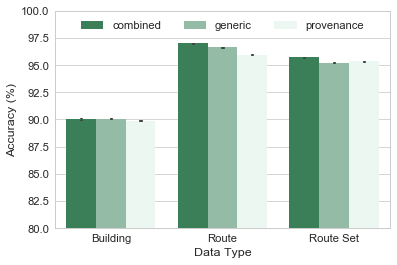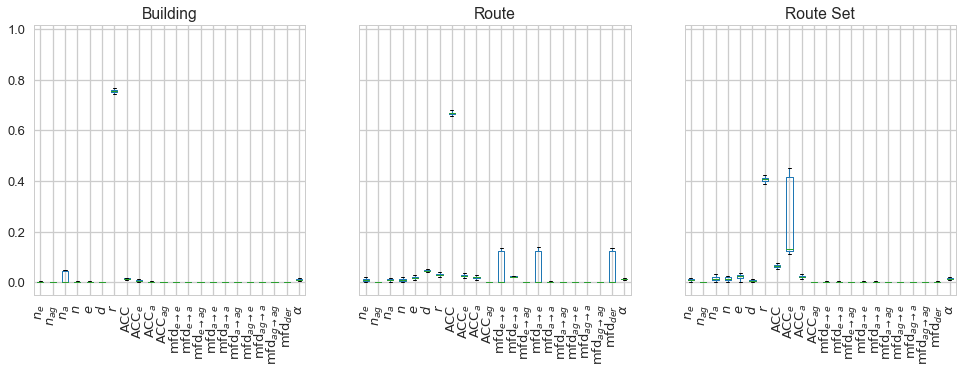# Application 2: CollabMap Data Quality¶

Assessing the quality of crowdsourced data in CollabMap from their provenance

• Goal: To determine if the provenance network analytics method can identify trustworthy data (i.e. buildings, routes, and route sets) contributed by crowd workers in CollabMap.
• Classification labels: $\mathcal{L} = \left\{ \textit{trusted}, \textit{uncertain} \right\}$.
• Training data:
• Buildings: 5175
• Routes: 4710
• Route sets: 4997

The CollabMap dataset is provided in the collabmap/depgraphs.csv file, each row corresponds to a building, route, or route sets created in the application:

• id: the identifier of the data entity (i.e. building/route/route set).
• trust_value: the beta trust value calculated from the votes for the data entity.
• The remaining columns provide the provenance network metrics calculated from the dependency provenance graph of the entity.
In :
import pandas as pd

In :
df = pd.read_csv("collabmap/depgraphs.csv", index_col='id')

Out:
trust_value entities agents activities nodes edges diameter assortativity acc acc_e ... mfd_e_a mfd_e_ag mfd_a_e mfd_a_a mfd_a_ag mfd_ag_e mfd_ag_a mfd_ag_ag mfd_der powerlaw_alpha
id
Route41053.0 0.833333 9 0 6 15 26 3 -0.272207 0.891091 0.809409 ... 1 0 2 0 0 0 0 0 2 -1.00000
RouteSet9042.1 0.600000 6 0 3 9 15 2 -0.412974 0.879630 0.847222 ... 1 0 1 0 0 0 0 0 1 -1.00000
Building19305.0 0.428571 6 0 4 10 13 2 -0.527046 0.901235 0.822222 ... 1 0 1 0 0 0 0 0 1 3.19876
Building1136.0 0.428571 6 0 4 10 13 2 -0.527046 0.901235 0.822222 ... 1 0 1 0 0 0 0 0 1 3.19876
Building24156.0 0.833333 9 0 5 14 24 3 -0.363937 0.838034 0.757639 ... 2 0 2 2 0 0 0 0 2 -1.00000

5 rows × 23 columns

In :
df.describe()

Out:
trust_value entities agents activities nodes edges diameter assortativity acc acc_e ... mfd_e_a mfd_e_ag mfd_a_e mfd_a_a mfd_a_ag mfd_ag_e mfd_ag_a mfd_ag_ag mfd_der powerlaw_alpha
count 14882.000000 14882.000000 14882.0 14882.000000 14882.000000 14882.000000 14882.000000 14882.000000 14882.000000 14882.000000 ... 14882.000000 14882.0 14882.000000 14882.000000 14882.0 14882.0 14882.0 14882.0 14882.000000 14882.000000
mean 0.766706 13.384693 0.0 6.793375 20.178067 39.118868 2.771267 -0.363791 0.806123 0.762426 ... 1.545424 0.0 1.742575 0.987166 0.0 0.0 0.0 0.0 1.802782 -0.226061
std 0.115301 17.165677 0.0 7.247706 24.147888 59.648535 0.917298 0.238658 0.203627 0.200090 ... 1.044079 0.0 1.012615 1.391763 0.0 0.0 0.0 0.0 0.938974 1.590865
min 0.153846 2.000000 0.0 0.000000 2.000000 1.000000 1.000000 -1.000000 0.000000 0.000000 ... 0.000000 0.0 0.000000 0.000000 0.0 0.0 0.0 0.0 1.000000 -1.000000
25% 0.750000 5.000000 0.0 2.000000 7.000000 10.000000 2.000000 -0.500000 0.820309 0.757639 ... 1.000000 0.0 1.000000 0.000000 0.0 0.0 0.0 0.0 1.000000 -1.000000
50% 0.800000 9.000000 0.0 5.000000 14.000000 24.000000 3.000000 -0.330835 0.849790 0.809409 ... 1.000000 0.0 2.000000 0.000000 0.0 0.0 0.0 0.0 2.000000 -1.000000
75% 0.833333 14.000000 0.0 9.000000 22.000000 40.000000 3.000000 -0.251256 0.880083 0.854159 ... 2.000000 0.0 2.000000 2.000000 0.0 0.0 0.0 0.0 2.000000 -1.000000
max 0.965517 178.000000 0.0 70.000000 248.000000 706.000000 13.000000 0.494008 1.000000 1.000000 ... 13.000000 0.0 12.000000 13.000000 0.0 0.0 0.0 0.0 12.000000 4.674298

8 rows × 23 columns

## Labelling data¶

Based on its trust value, we categorise the data entity into two sets: trusted and uncertain. Here, the threshold for the trust value, whose range is [0, 1], is chosen to be 0.75.

In :
trust_threshold = 0.75
df['label'] = df.apply(lambda row: 'Trusted' if row.trust_value >= trust_threshold else 'Uncertain', axis=1)
df.head()  # The new label column is the last column below

Out:
trust_value entities agents activities nodes edges diameter assortativity acc acc_e ... mfd_e_ag mfd_a_e mfd_a_a mfd_a_ag mfd_ag_e mfd_ag_a mfd_ag_ag mfd_der powerlaw_alpha label
id
Route41053.0 0.833333 9 0 6 15 26 3 -0.272207 0.891091 0.809409 ... 0 2 0 0 0 0 0 2 -1.00000 Trusted
RouteSet9042.1 0.600000 6 0 3 9 15 2 -0.412974 0.879630 0.847222 ... 0 1 0 0 0 0 0 1 -1.00000 Uncertain
Building19305.0 0.428571 6 0 4 10 13 2 -0.527046 0.901235 0.822222 ... 0 1 0 0 0 0 0 1 3.19876 Uncertain
Building1136.0 0.428571 6 0 4 10 13 2 -0.527046 0.901235 0.822222 ... 0 1 0 0 0 0 0 1 3.19876 Uncertain
Building24156.0 0.833333 9 0 5 14 24 3 -0.363937 0.838034 0.757639 ... 0 2 2 0 0 0 0 2 -1.00000 Trusted

5 rows × 24 columns

Having used the trust valuue to label all the data entities, we remove the trust_value column from the data frame.

In :
# We will not use trust value from now on
df.drop('trust_value', axis=1, inplace=True)
df.shape  # the dataframe now have 23 columns (22 metrics + label)

Out:
(14882, 23)

## Filtering data¶

We split the dataset into three: buildings, routes, and route sets.

In :
df_buildings = df.filter(like="Building", axis=0)
df_routes = df.filter(regex="^Route\d", axis=0)
df_routesets = df.filter(like="RouteSet", axis=0)
df_buildings.shape, df_routes.shape, df_routesets.shape  # The number of data points in each dataset

Out:
((5175, 23), (4997, 23), (4710, 23))

## Balancing Data¶

This section explore the balance of each of the three datasets and balance them using the SMOTE Oversampling Method.

In :
from analytics import balance_smote


### Buildings¶

In :
df_buildings.label.value_counts()

Out:
Trusted      4491
Uncertain     684
Name: label, dtype: int64

Balancing the building dataset:

In :
df_buildings = balance_smote(df_buildings)

Original data shapes: (5175, 22) (5175,)
Balanced data shapes: (8982, 22) (8982,)


### Routes¶

In :
df_routes.label.value_counts()

Out:
Trusted      3908
Uncertain    1089
Name: label, dtype: int64

Balancing the route dataset:

In :
df_routes = balance_smote(df_routes)

Original data shapes: (4997, 22) (4997,)
Balanced data shapes: (7816, 22) (7816,)


### Route Sets¶

In :
df_routesets.label.value_counts()

Out:
Trusted      3019
Uncertain    1691
Name: label, dtype: int64

Balancing the route set dataset:

In :
df_routesets = balance_smote(df_routesets)

Original data shapes: (4710, 22) (4710,)
Balanced data shapes: (6038, 22) (6038,)


## Cross Validation¶

We now run the cross validation tests on the three balanaced datasets (df_buildings, df_routes, and df_routesets) using all the features (combined), only the generic network metrics (generic), and only the provenance-specific network metrics (provenance). Please refer to Cross Validation Code.ipynb for the detailed description of the cross validation code.

In :
from analytics import test_classification


### Building Classification¶

We test the classification of buildings, collect individual accuracy scores results and the importance of every feature in each test in importances (both are Pandas Dataframes). These two tables will also be used to collect data from testing the classification of routes and route sets later.

In :
# Cross validation test on building classification
res, imps = test_classification(df_buildings)

# adding the Data Type column
res['Data Type'] = 'Building'
imps['Data Type'] = 'Building'

# storing the results and importance of features
results = res
importances = imps

# showing a few newest rows
results.tail()

Accuracy: 90.03% ±0.0576 <-- combined
Accuracy: 90.06% ±0.0557 <-- generic
Accuracy: 89.90% ±0.0581 <-- provenance

Out:
Accuracy Metrics Data Type
2995 0.891982 provenance Building
2996 0.885301 provenance Building
2997 0.918708 provenance Building
2998 0.887528 provenance Building
2999 0.902004 provenance Building

### Route Classification¶

In :
# Cross validation test on route classification
res, imps = test_classification(df_routes)

# adding the Data Type column
res['Data Type'] = 'Route'
imps['Data Type'] = 'Route'

# storing the results and importance of features
results = results.append(res, ignore_index=True)
importances = importances.append(imps, ignore_index=True)

# showing a few newest rows
results.tail()

Accuracy: 96.98% ±0.0368 <-- combined
Accuracy: 96.63% ±0.0404 <-- generic
Accuracy: 95.97% ±0.0433 <-- provenance

Out:
Accuracy Metrics Data Type
5995 0.943734 provenance Route
5996 0.960358 provenance Route
5997 0.964194 provenance Route
5998 0.957692 provenance Route
5999 0.958974 provenance Route

### Route Set Classification¶

In :
# Cross validation test on route classification
res, imps = test_classification(df_routesets)

# adding the Data Type column
res['Data Type'] = 'Route Set'
imps['Data Type'] = 'Route Set'

# storing the results and importance of features
results = results.append(res, ignore_index=True)
importances = importances.append(imps, ignore_index=True)

# showing a few newest rows
results.tail()

Accuracy: 95.70% ±0.0493 <-- combined
Accuracy: 95.20% ±0.0526 <-- generic
Accuracy: 95.34% ±0.0500 <-- provenance

Out:
Accuracy Metrics Data Type
8995 0.950331 provenance Route Set
8996 0.947020 provenance Route Set
8997 0.968543 provenance Route Set
8998 0.965232 provenance Route Set
8999 0.950166 provenance Route Set

### Saving experiments' results (optional)¶

Optionally, we can save the test results to save time the next time we want to re-explore them:

In :
results.to_pickle("collabmap/results.pkl")
importances.to_pickle("collabmap/importances.pkl")


Next time, we can reload the results as follows:

In :
import pandas as pd
results.shape, importances.shape  # showing the shape of the data (for checking)

Out:
((9000, 3), (3000, 23))

### Charting the accuracy scores¶

In :
%matplotlib inline
import seaborn as sns
sns.set_style("whitegrid")
sns.set_context("paper", font_scale=1.4)


Converting the accuracy score from [0, 1] to percentage, i.e [0, 100]:

In :
results.Accuracy = results.Accuracy * 100

Out:
Accuracy Metrics Data Type
0 90.444444 combined Building
1 89.866370 combined Building
2 90.311804 combined Building
3 90.200445 combined Building
4 88.307350 combined Building
In :
from matplotlib.font_manager import FontProperties
fontP = FontProperties()
fontP.set_size(12)

In :
pal = sns.light_palette("seagreen", n_colors=3, reverse=True)
plot = sns.barplot(x="Data Type", y="Accuracy", hue='Metrics', palette=pal, errwidth=1, capsize=0.02, data=results)
plot.set_ylim(80, 100)
plot.legend(loc='upper center', bbox_to_anchor=(0.5, 1.0), ncol=3)
plot.set_ylabel('Accuracy (%)')

Out:
<matplotlib.text.Text at 0x109c1acf8>Saving the chart above to Fig4.eps to be included in the paper:

In :
plot.figure.savefig("figures/Fig4.eps")


### Charting the importance of features¶

In this section, we explore the relevance of each features in classifying the data quality of CollabMap buildings, routes, and route sets. To do so, we analyse the feature importance values provided by the decision tree training done above - the importances data frame.

In :
import numpy as np

In :
# Rename the columns with Math notation for consistency with the metrics symbols in the paper
feature_name_maths_mapping = {
"entities": "$n_e$", "agents": "$n_{ag}$", "activities": "$n_a$", "nodes": "$n$", "edges": "$e$",
"diameter": "$d$", "assortativity": "$r$", "acc": "$\\mathsf{ACC}$",
"acc_e": "$\\mathsf{ACC}_e$",  "acc_a": "$\\mathsf{ACC}_a$",  "acc_ag": "$\\mathsf{ACC}_{ag}$",
"mfd_e_e": "$\\mathrm{mfd}_{e \\rightarrow e}$", "mfd_e_a": "$\\mathrm{mfd}_{e \\rightarrow a}$",
"mfd_e_ag": "$\\mathrm{mfd}_{e \\rightarrow ag}$", "mfd_a_e": "$\\mathrm{mfd}_{a \\rightarrow e}$",
"mfd_a_a": "$\\mathrm{mfd}_{a \\rightarrow a}$", "mfd_a_ag": "$\\mathrm{mfd}_{a \\rightarrow ag}$",
"mfd_ag_e": "$\\mathrm{mfd}_{ag \\rightarrow e}$", "mfd_ag_a": "$\\mathrm{mfd}_{ag \\rightarrow a}$",
"mfd_ag_ag": "$\\mathrm{mfd}_{ag \\rightarrow ag}$", "mfd_der": "$\\mathrm{mfd}_\\mathit{der}$", "powerlaw_alpha": "$\\alpha$"
}
importances.rename(columns=feature_name_maths_mapping, inplace=True)

In :
grouped =importances.groupby("Data Type")  # Grouping the importance values by data type

In :
sns.set_context("talk")
grouped.boxplot(figsize=(16, 5), layout=(1, 3), rot=90)

Out:
Building          Axes(0.1,0.15;0.235294x0.75)
Route        Axes(0.382353,0.15;0.235294x0.75)
Route Set    Axes(0.664706,0.15;0.235294x0.75)
dtype: objectThe charts above show us the relevance of each feature in classifying the quality of CollabMap buildings, routes, and route sets. Next, we find the three most relevant features for each data type to report in the paper.

In :
# Calculate the mean importance of each feature for each data type
imp_means = grouped.mean()

In :
pd.DataFrame(
{row_name: row.sort_values(ascending=False)[:3].index.get_values()
for row_name, row in imp_means.iterrows()
}
)

Out:
Building Route Route Set
0 $r$ $\mathsf{ACC}$ $r$
1 $\mathsf{ACC}$ $d$ $\mathsf{ACC}_e$
2 $n_a$ $\mathrm{mfd}_\mathit{der}$ $e$

The table above shows the most important metrics as reported by the decision tree classifiers during their training for each dataset.

### Retest the classifications using minimal sets of features (Extra)¶

Armed with the knowledge of the three most important features in each experiment, we re-run the experiments using only those.

In :
from analytics import cv_test

In :
res, imps = cv_test(df_buildings[['assortativity', 'acc', 'activities']], df_buildings.label, test_id="Building")

Accuracy: 89.96% ±0.0570 <-- Building

In :
res, imps = cv_test(df_routes[['acc', 'diameter', 'mfd_der']], df_routes.label, test_id="Route")

Accuracy: 95.09% ±0.0455 <-- Route

In :
res, imps = cv_test(df_routesets[['assortativity', 'acc_e', 'entities']], df_routesets.label, test_id="Routeset")

Accuracy: 95.05% ±0.0541 <-- Routeset


As shown above, using only three metrics from each dataset, we can still achieve a high level of accuracy from the classifiers: 90%, 95%, and 95% for buildings, routes, and route sets, respectively. The performance is very close to that when all the metrics were used (90%, 97%, 96%, respectively). This shows that, in certain applications, we can select a smaller set of metrics for classification based on the relevancy analysis above to reduce the computational cost without significantly affecting the classification performance.